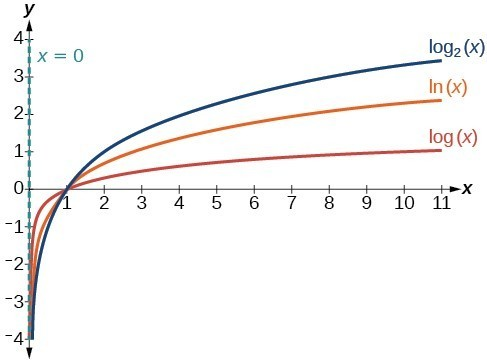## Graph logarithmic functions

Now that we have a feel for the set of values for which a logarithmic function is defined, we move on to graphing logarithmic functions. The family of logarithmic functions includes the parent function $y={\mathrm{log}}_{b}\left(x\right)$ along with all its transformations: shifts, stretches, compressions, and reflections.

We begin with the parent function $y={\mathrm{log}}_{b}\left(x\right)$. Because every logarithmic function of this form is the inverse of an exponential function with the form $y={b}^{x}$, their graphs will be reflections of each other across the line $y=x$. To illustrate this, we can observe the relationship between the input and output values of $y={2}^{x}$ and its equivalent $x={\mathrm{log}}_{2}\left(y\right)$ in the table below.

 x –3 –2 –1 0 1 2 3 ${2}^{x}=y$ $\frac{1}{8}$ $\frac{1}{4}$ $\frac{1}{2}$ 1 2 4 8 ${\mathrm{log}}_{2}\left(y\right)=x$ –3 –2 –1 0 1 2 3

Using the inputs and outputs from the table above, we can build another table to observe the relationship between points on the graphs of the inverse functions $f\left(x\right)={2}^{x}$ and $g\left(x\right)={\mathrm{log}}_{2}\left(x\right)$.

 $f\left(x\right)={2}^{x}$ $\left(-3,\frac{1}{8}\right)$ $\left(-2,\frac{1}{4}\right)$ $\left(-1,\frac{1}{2}\right)$ $\left(0,1\right)$ $\left(1,2\right)$ $\left(2,4\right)$ $\left(3,8\right)$ $g\left(x\right)={\mathrm{log}}_{2}\left(x\right)$ $\left(\frac{1}{8},-3\right)$ $\left(\frac{1}{4},-2\right)$ $\left(\frac{1}{2},-1\right)$ $\left(1,0\right)$ $\left(2,1\right)$ $\left(4,2\right)$ $\left(8,3\right)$

As we’d expect, the x– and y-coordinates are reversed for the inverse functions. The figure below shows the graph of f and g.

Figure 2. Notice that the graphs of $f\left(x\right)={2}^{x}$ and $g\left(x\right)={\mathrm{log}}_{2}\left(x\right)$ are reflections about the line = x.

Observe the following from the graph:

• $f\left(x\right)={2}^{x}$ has a y-intercept at $\left(0,1\right)$ and $g\left(x\right)={\mathrm{log}}_{2}\left(x\right)$ has an x-intercept at $\left(1,0\right)$.
• The domain of $f\left(x\right)={2}^{x}$, $\left(-\infty ,\infty \right)$, is the same as the range of $g\left(x\right)={\mathrm{log}}_{2}\left(x\right)$.
• The range of $f\left(x\right)={2}^{x}$, $\left(0,\infty \right)$, is the same as the domain of $g\left(x\right)={\mathrm{log}}_{2}\left(x\right)$.

### A General Note: Characteristics of the Graph of the Parent Function, f(x) = logb(x)

For any real number x and constant > 0, $b\ne 1$, we can see the following characteristics in the graph of $f\left(x\right)={\mathrm{log}}_{b}\left(x\right)$:

• one-to-one function
• vertical asymptote: = 0
• domain: $\left(0,\infty \right)$
• range: $\left(-\infty ,\infty \right)$
• x-intercept: $\left(1,0\right)$ and key point $\left(b,1\right)$
• y-intercept: none
• increasing if $b>1$
• decreasing if 0 < < 1

Figure 3 shows how changing the base b in $f\left(x\right)={\mathrm{log}}_{b}\left(x\right)$ can affect the graphs. Observe that the graphs compress vertically as the value of the base increases. (Note: recall that the function $\mathrm{ln}\left(x\right)$ has base $e\approx \text{2}.\text{718.)}$Figure 4. The graphs of three logarithmic functions with different bases, all greater than 1.

### How To: Given a logarithmic function with the form $f\left(x\right)={\mathrm{log}}_{b}\left(x\right)$, graph the function.

1. Draw and label the vertical asymptote, x = 0.
2. Plot the x-intercept, $\left(1,0\right)$.
3. Plot the key point $\left(b,1\right)$.
4. Draw a smooth curve through the points.
5. State the domain, $\left(0,\infty \right)$, the range, $\left(-\infty ,\infty \right)$, and the vertical asymptote, x = 0.

### Example 3: Graphing a Logarithmic Function with the Form $f\left(x\right)={\mathrm{log}}_{b}\left(x\right)$.

Graph $f\left(x\right)={\mathrm{log}}_{5}\left(x\right)$. State the domain, range, and asymptote.

### Solution

Before graphing, identify the behavior and key points for the graph.

• Since = 5 is greater than one, we know the function is increasing. The left tail of the graph will approach the vertical asymptote = 0, and the right tail will increase slowly without bound.
• The x-intercept is $\left(1,0\right)$.
• The key point $\left(5,1\right)$ is on the graph.
• We draw and label the asymptote, plot and label the points, and draw a smooth curve through the points.

Figure 5. The domain is $\left(0,\infty \right)$, the range is $\left(-\infty ,\infty \right)$, and the vertical asymptote is x = 0.

### Try It 3

Graph $f\left(x\right)={\mathrm{log}}_{\frac{1}{5}}\left(x\right)$. State the domain, range, and asymptote.

Solution